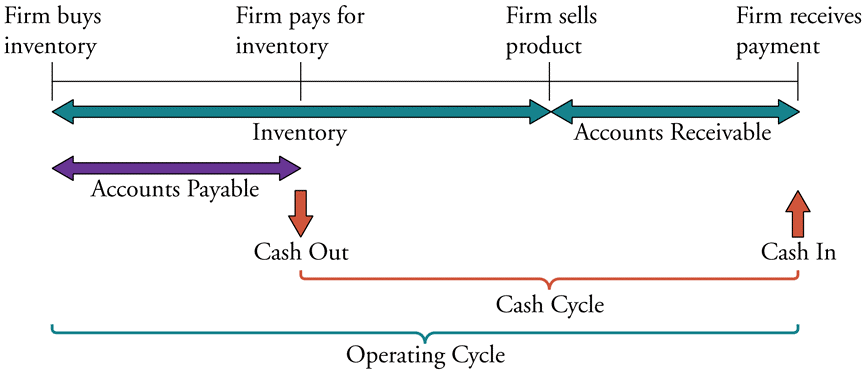# Fight Finance

#### CoursesTagsRandomAllRecentScores

The required return of a project is 10%, given as an effective annual rate.

What is the payback period of the project in years?

Assume that the cash flows shown in the table are received smoothly over the year. So the $121 at time 2 is actually earned smoothly from t=1 to t=2.  Project Cash Flows Time (yrs) Cash flow ($) 0 -100 1 11 2 121

Due to floods overseas, there is a cut in the supply of the mineral iron ore and its price increases dramatically. An Australian iron ore mining company therefore expects a large but temporary increase in its profit and cash flows. The mining company does not have any positive NPV projects to begin, so what should it do? Select the most correct answer.

A project's net present value (NPV) is negative. Select the most correct statement.

Will the price of a call option on equity or if the standard deviation of returns (risk) of the underlying shares becomes higher?

In Australia in the 1980's, inflation was around 8% pa, and residential mortgage loan interest rates were around 14%.

In 2013, inflation was around 2.5% pa, and residential mortgage loan interest rates were around 4.5%.

If a person can afford constant mortgage loan payments of \$2,000 per month, how much more can they borrow when interest rates are 4.5% pa compared with 14.0% pa?

Give your answer as a proportional increase over the amount you could borrow when interest rates were high $(V_\text{high rates})$, so:

$$\text{Proportional increase} = \dfrac{V_\text{low rates}-V_\text{high rates}}{V_\text{high rates}}$$

Assume that:

• Interest rates are expected to be constant over the life of the loan.
• Loans are interest-only and have a life of 30 years.
• Mortgage loan payments are made every month in arrears and all interest rates are given as annualised percentage rates (APR's) compounding per month.

Discounted cash flow (DCF) valuation prices assets by finding the present value of the asset's future cash flows. The single cash flow, annuity, and perpetuity equations are very useful for this.

Which of the following equations is the 'perpetuity with growth' equation?

How many years will it take for an asset's price to double if the price grows by 10% pa?

Which one of the below option and futures contracts gives the possibility of potentially unlimited gains?

Which of the following is NOT the Australian central bank’s responsibility?

The below diagram shows a firm’s cash cycle.Which of the following statements about companies’ cash cycle is NOT correct?